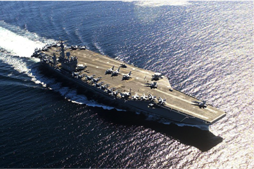Forces and Motion

Equation

velocity =

acceleration x time

v = at

1.4

Equation

final velocity = initial velocity + acceleration x time

vf = v+ at

1.5

Equation

average velocity = initial velocity + final velocity ÷ two

vave = (vi + vf) / 2

1.6

Equation

distance traveled  =  ½ x acceleration x times squared

d = ½ at2

1.7

Acceleration

Example QuestionIf a plane on an aircraft carrier accelerates from rest to 90 m/s in 3 seconds, its average acceleration from Eqn 1.3 is:

a = v/t = (90 m/s)/3 s = 30 m/s2

The speed or velocity that the plane is moving can also be calculated knowing its acceleration and the amount of time it has been accelerating. Just rearrange equation (1.3) to get:

velocity = acceleration x time    v = at

If an object was already moving and then accelerated, its final velocity, vf, is:

Or more generally,    final velocity = initial velocity + acceleration x time    vf = vi + at

In many problems, such as this one, the initial velocity, vi, is zero.

Of course, the plane needs to accelerate to 90 m/s this speed (if that is what it takes to become airborne) before it goes careening off the bow of the aircraft carrier into the ocean. So, you might ask, how far has the plane traveled in the 3 seconds it took for it to accelerate to 90 m/s? We can figure this out by mathematically juggling things you already know.

Since from Eqn 1.1: v = d/t, then d = vt or distance equals velocity x time, which is true for the average velocity. By definition the average velocity is found by adding initial and final velocities and dividing by 2 (or multiplying by ½):

vave = ½ (vi + vf)

d = ½ (vi + vf) t

Now we substitute what vf  is from equation 1.5 into this to solve for distance traveled in terms of time and acceleration:

d = ½ (vi + vi + at) t

now add the two vi together:

d = ½ (2vi + at) t

now multiply each term by t:

d = ½(2vi t+ at2)

finally, divide each term by 2:

d = vi t+ ½at2

and if vi is zero, then

distance traveled  =  ½ x acceleration x times squared   d = ½ at2

So, how far did the plane travel in 3 seconds?

d = ½ at2  =  ½ x 30 x 32   =  15 m/s2 x 9 s2  =  135 m

And how long are aircraft carriers? The largest, such as the USS Nimitz, is 333 m long, providing plenty of room to accelerate fast enough for a safe takeoff!

When you first glanced at this page and saw the equations you may have groaned that physics is difficult. But I hope that as you read through the equations you saw that each step is easy and logical. If so you are beginning to think like a physicist!